Latest Teaching jobs   »   Electric Current – Science Notes for...

# Electric Current – Science Notes for CTET 2020: FREE PDF## ELECTRIC CURRENT

Electric Current: The flow of electric charge is known as Electric Current, Electric current is carried by moving electrons through a conductor. Electric current flows in the opposite direction to the movement of electrons.

Expression of Electric Current:
Electric current is denoted by the letter ‘I’. Electric current is expressed by the rate of flow of electric charges. Rate of flow means, the amount of charge flowing through a particular area in unit time.

If a net electric charge (Q) flows through a cross-section of a conductor in time t, then,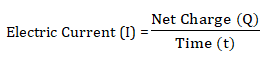Where, “I” is electric current, “Q” is a net charge and “t” is a time in second.

S.I. Unit of Electric Charge and Current:
• S.I. unit of electric charge is coulomb (C).
• One coulomb is nearly equal to 6 × 1018 electrons.
• S.I. unit of electric current is ampere (A).

Ampere: Ampere is the flow of electric charge through a surface at the rate of one coulomb per second. This means, if 1 coulomb of electric charge flows through a cross section for 1 second, it would be equal to 1 ampere.
Therefore, 1 A = 1 C/1 sec.

Ammeter: An apparatus to measure electric current in a circuit.,

Charge: Like mass, the charge is the fundamental property of matter. There are two types of charge:
(i) Positive charge: The charge acquired by a glass rod when rubbed with silk is called a positive charge.
(ii) Negative charge: the charge acquired by an ebonite rod when rubbed with wool is called negative charge.

Properties of Electric Charge:
(i) Unlike charges attract each other and like charges repel each other.
(ii) The force between two charges varies directly as the product of two charges and inversely as the square of the distance (r) between both charges (q1 and q2).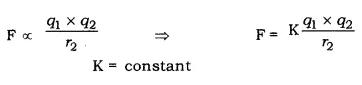S.I. unit of charge is coulomb (C).
1 coulomb = 1 ampere × 1 second.
1C = 1A × 1s
Thus, the quantity of charge which flows through a circuit when one ampere of current flows through it in one second is known as a 1-coulomb charge.

Electric Potential: The amount of electric potential energy at a point is called electric potential.

Potential Difference: The difference in the amount of electric potential energy between two points in an electric circuit is called electric potential difference.
Electric potential difference is known as voltage, which is equal to the amount of work done to move the unit charge between two points against static electric field.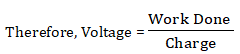Voltage or electric potential difference is denoted by V’.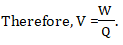S.I. Unit of Electric Potential Difference (Voltage).

Since, joule is the unit of work and Coulomb is the unit of charge, 1 volt of electric potential difference is equal to the 1 joule of work to be done to move a charge of 1 coulomb from one point to another in an electric circuit. Therefore,
1V = 1Joule/1Coulomb = 1J/1C
1V = 1JC-1

Voltmeter: An apparatus to measure the potential difference or electric potential difference between two points in an electric circuit.

Galvanometer: It is a device to detect current in an electric circuit.

Ohm’s Law:
Ohm’s Law states that the potential difference between two points is directly proportional to the electric current, at a constant temperature.
This means potential difference V varies as electric current.
V α I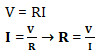Where, R is constant for the given conductor at a given temperature and is called resistance.

Resistance: Resistance is the property of conductor which resists the flow of electric current through it.
S.I. unit of resistance is ohm. Ohm is denoted ‘Q’.

1 Ohm: 1 ohm (Q) of resistance (R) is equal to the flow 1A of current through a conductor between two points having a potential difference equal to 1V.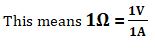From the expression of Ohm’s Law, electric current will decrease with an increase in resistance and vice versa. The graph of V (potential difference) versus I (electric current) is always a straight line.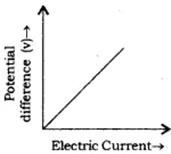Resistor: A component that is used to resist the flow of electric current in a circuit is called a resistor. In practical application, resistors are used to increase or decrease the electric current.

Variable Resistance: The component of an electric circuit which is used to regulate the current, without changing the voltage from the source, is called variable resistance.

Rheostat: This is a device which is used in a circuit to provide variable resistance.

Cause of Resistance in a Conductor:
• Flow of electrons in a conductor is electric current.
• The positive particles of conductor create hindrance to flow of electrons, because of attraction between them; this hindrance is the cause of resistance in the flow of electricity.

Factors on Which Resistance of a Conductor Depends:

(i) Nature of Material: Silver is the best conductor of electricity. While some other materials create more hindrance in the flow of electric current, i.e. flow of electrons through them. Such materials are called bad conductors. Bad conductor is also known as insulators.

(ii) Length of Conductor: Resistance (R) is directly proportional to the length of the conductor. This means, resistance increases with increase in length of the conductor. This is the cause that long electric wires create more resistance to the electric current. Thus, Resistance (R) α length of conductor (l).

(iii) Area of Cross Section: Resistance R is inversely proportional to the area of cross section (A) of the conductor. This means R will decrease with an increase in the area of conductor and vice versa. More area of conductor facilitates the flow of electric current through more area and thus, decreases the resistance.

Resistivity: It is defined as the resistance offered by a cube of a material of side 1m when current flow perpendicular to its opposite faces. Its S.I. unit is ohm-meter (Ωm).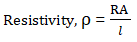Resistivity is also known as specific resistance.
Resistivity depends on the nature of the material of the conductor.

I. Resistors in Series:
When resistors are joined from end to end, it is called in series.
The total resistance of the system is equal to the sum of the resistance of all the resistors in the system.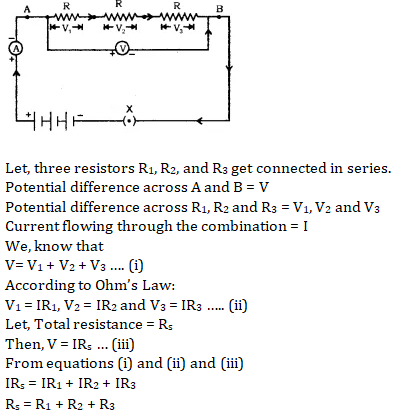When the resistors are connected in series, the current flowing through each resistor is the same and is equal to the total current.

II. Resistors in Parallel:
When resistors are joined in parallel, the reciprocal of the total resistance of the system is equal to the sum of reciprocal of the resistance of resistors.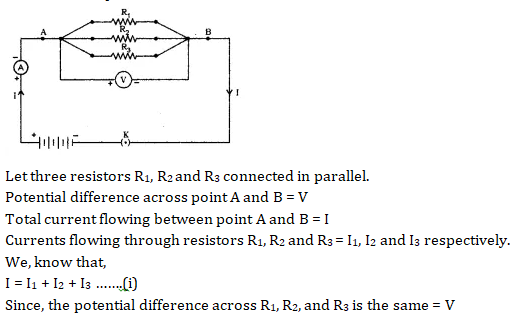According to Ohm’s Law,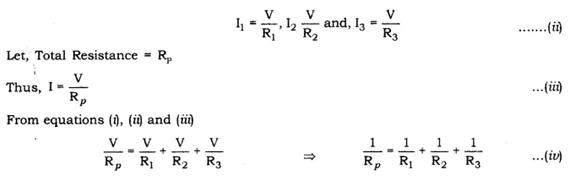In parallel combination, the potential difference across each resistor is the same and is equal to the total potential difference.

•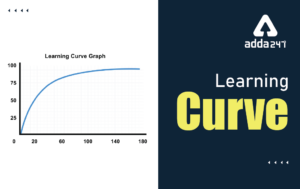Learning Curve - What is Learning Curve?...
•रस - परिभाषा, भे�...
•पठन कौशल- परिभा�...
•अलंकार - परिभाष�...
•Indian States and Capitals 2022: 28 Stat...
•New Education Policy(NEP) PDF - नई �...